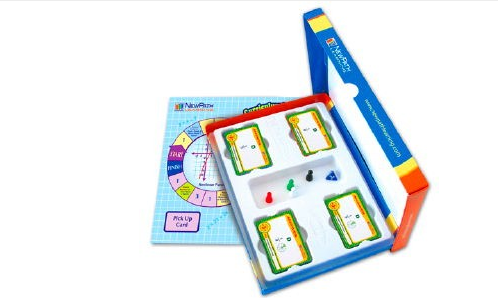# Tất cả sản phẩm# Grade 7 Math Curriculum Mastery® Game - Study-Group Edition

Mã sản phẩm: 23-7401 Lược xem: 637

Liên hệ

Includes one double-sided game board, a blended set of 250 cards representing a cross-section of the topics represented in the Class-Pack Edition (23-7001), materials for four students, and trial online subscription. Topics include:

1. Organizing Data
2. Analyzing, Graphing & Displaying Data
3. Introduction to Algebra
4. Equations & Inequalities
5. Using Integers
6. Rational & Irrational Numbers
7. Decimal Operations
8. Exponents, Factors & Fractions
9. Fraction Operations
10. Numerical Proportions
11. Geometric Proportions
12. Introduction to Percent
13. Applying Percents
14. Plane Figures: Lines & Angles
15. Plane Figures: Closed Figure Relationships
16. Measurement, Perimeter & Circumference
17. Area & Surface Area
18. The Pythagorean Theorem
19. Finding Volume
20. Introduction to Probability
21. Using Probability
22. Algebraic Equations
23. Algebraic Inequalities
24. Introduction to Functions
25. Nonlinear Functions & Set Theory